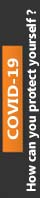2019-01-25T10:18:42+00:00 Assignments

This paper concentrates on the primary theme of Advanced Concepts of Finance in which you have to explain and evaluate its intricate aspects in detail. In addition to this, this paper has been reviewed and purchased by most of the students hence; it has been rated 4.8 points on the scale of 5 points. Besides, the price of this paper starts from £ 40. For more details and full access to the paper, please refer to the site.

QUESTION ONE

1. Value Of One Year European Option For One Year

Current price = £ 200

242 = (242 – 200 = 42)

220

200                                          198 = (198 – 200, 0)

180

162 =    (162 – 200, 0)

Put option value = (St – x, 0) n

Rate = 6% for 6 months which is be taken as 12% for the whole year

= 42 × 1.12)*^-1

= £ 37.5

1. Assuming it is an American option

Current price = £ 200

242 = (242 – 200 = 42)

220

200                                          198 = (198 – 200, 0)

180

162 =    (162 – 200, 0)

Put option value = (St – x, 0) n

Therefore, value = (42 ×1.06^-2) + (220-200×1.06^-1)

= 37.38 + 18.87

= £ 56.25

QUESTION TWO

Value of a European put option = P 0 = X e –rT [1 – N (d2)] – S 0 [1 – N (d 1)]

For this European put option:

S = \$20

X = 10 – 30

T = 2

e = 2.71828

PV = K e^ -rT (20 * 2.71828^-2*0.04) = 18.46

K ≥ PEU ≥ Max [0, PVOT (K) – FPO, T (S)]

= Max [0, PVOT (K) – S (0) + D]

At S = 10, K = 8.46 – (10*0.05*0.2) = 0.1 = 8.36

 S 10 11 12 13 14 15 16 17 18 19 20 21 22 23 24 25 26 27 28 29 30 Put Value K 8.4 7.4 6.4 5.3 4.3 3.3 2.3 1.3 0.3 0 0 0 0 0 0 0 0 0 0 0 0

An existence of a point in which the option price is lower than the intrinsic value indicates that there exist an arbitrage opportunity which arises from exercising an option after buying it at the bargaining price. In this European put option, there is a region where the option trades for less than its intrinsic value for un-discernable length of time. the existence of low intrinsic value than the option price can be attribute to point in which there was no transaction occurring or the market was closed. On addition to this, the option is in some region out of money because it has worthless expiration risk, when it tends to be relatively inexpensive (Whaley, 2012).

Dividend PV = (0.05 * 20 * 0.2 *2.71828^-2*0.04)

= 0.184

Strike price = 20 *2.71828^-2*0.04 = 18.46 – 0.184

Current price = £ 18.65

QUESTION THREE

1. Value of option of cancelling the project

Expected cash flow

37.5

25

18.75

Expected cash flow = (37.5 *0.5*4) + (18.75 * 0.25*4) = £ 93.75 million

From 4 million number of barrels 4 million.

NPV of the project is 93.75 * (1.01^-1) – 110 = -£ 17.17 million

The firm should not invest in this project.

Value of cancelling the option

(85 * 1.01^ -1) – 110 = -£25.84 million

1. Without political donation

Probability of opposition winning = 20%

If they win and oil goes up revenue = (0.8 * 37.5 * 4000000) = £ 120000000

Tax = 120000000 – (0.8 * 120000000) = 96000000 – 110000000 = loss 14 million

They should not go ahead without political donation

1. c) The firm will be willing to donate a maximum of 12 million, which is equivalent to the value of the additional tax

QUESTION FOUR

1. Interest parity theory holds that the interest rate differential two countries should be equal to the differential between the spot exchange rate and forward exchange rate.

Therefore futures price of one R\$ be in US\$ according to the covered interest rate, parity theorem is:

= spot rate × (1 + oversee interest rate)/ (1 + domestic country interest rate

= 1.8 × [(1 + 10%)/ (1 + 1%)]

= R 1.96 per US \$

1. The arbitrage opportunity exists in the case because the forward exchange rate does not incorporate the two countries interest rate difference. This means that there exist an interest rate advantage if the money is borrowed from US (which is characterized by a low interest rate) to be invested in Brazil (which offers a high interest rate).
2. A table of necessary actions to get R \$ 12 million in one year at no cost (Whaley, 2012)
 Action now Cash flow now in Real Cash Flow In Real In The Future Less Borrowed And  Interest Borrow US \$ 254.24 million at interest rate of 1% 300.0032 million 315.00336 million 354 -(254.24 * 1.18 * 1.01)million one-year forward contract for the purchase of US \$ 254.24 million at 1 US dollar per R\$ 1.18 0 0 0 Total 12.00 Million

Reference list

Whaley, R. (2012). Derivatives markets, valuation, and risk management. Hoboken, N.J: Wiley.

0% Plagiarism Guaranteed & Custom Written, Tailored to your instructions

International House, 12 Constance Street, London, United Kingdom,
E16 2DQ

## STILL NOT CONVINCED?

We've produced some samples of what you can expect from our Academic Writing Service - these are created by our writers to show you the kind of high-quality work you'll receive. Take a look for yourself!FLAT 25% OFF ON EVERY ORDER.Use "FLAT25" as your promo code during checkout